### The tidygraph package

The tidygraph package is part of the tidyverse and combines very well with other packages like dplyr or tidytext. In this tutorial, we will use dplyr and tidygraph to start handling network data:

``````library(dplyr)
library(tidygraph)``````

After loading the packages, we can use the functions of tidygraph. These functions can be used to read network data from files, to manipulate that data, and also to analyze it and visualize it. Tidygraph allows you to generate random networks or fixed network structures from a variety of methods. For example, here we build a ring graph, which takes the form of a single cycle with N nodes:

``````ringgraph <- create_ring(12)
ringgraph``````
``````## # A tbl_graph: 12 nodes and 12 edges
## #
## # An undirected simple graph with 1 component
## #
## # Node Data: 12 x 0 (active)
## #
## # Edge Data: 12 x 2
##    from    to
##   <int> <int>
## 1     1     2
## 2     2     3
## 3     3     4
## # … with 9 more rows``````

When you call ringgraph above, you see that the output is of the type tbl_graph, a special kind of tibble that contains graph information. It has 12 nodes and edges, and you get some information on the endpoints of few edges of the graph.

You can have a better idea of the graph if you run the plot() function over it, which automatically uses parts of another package called igraph:

``plot(ringgraph)``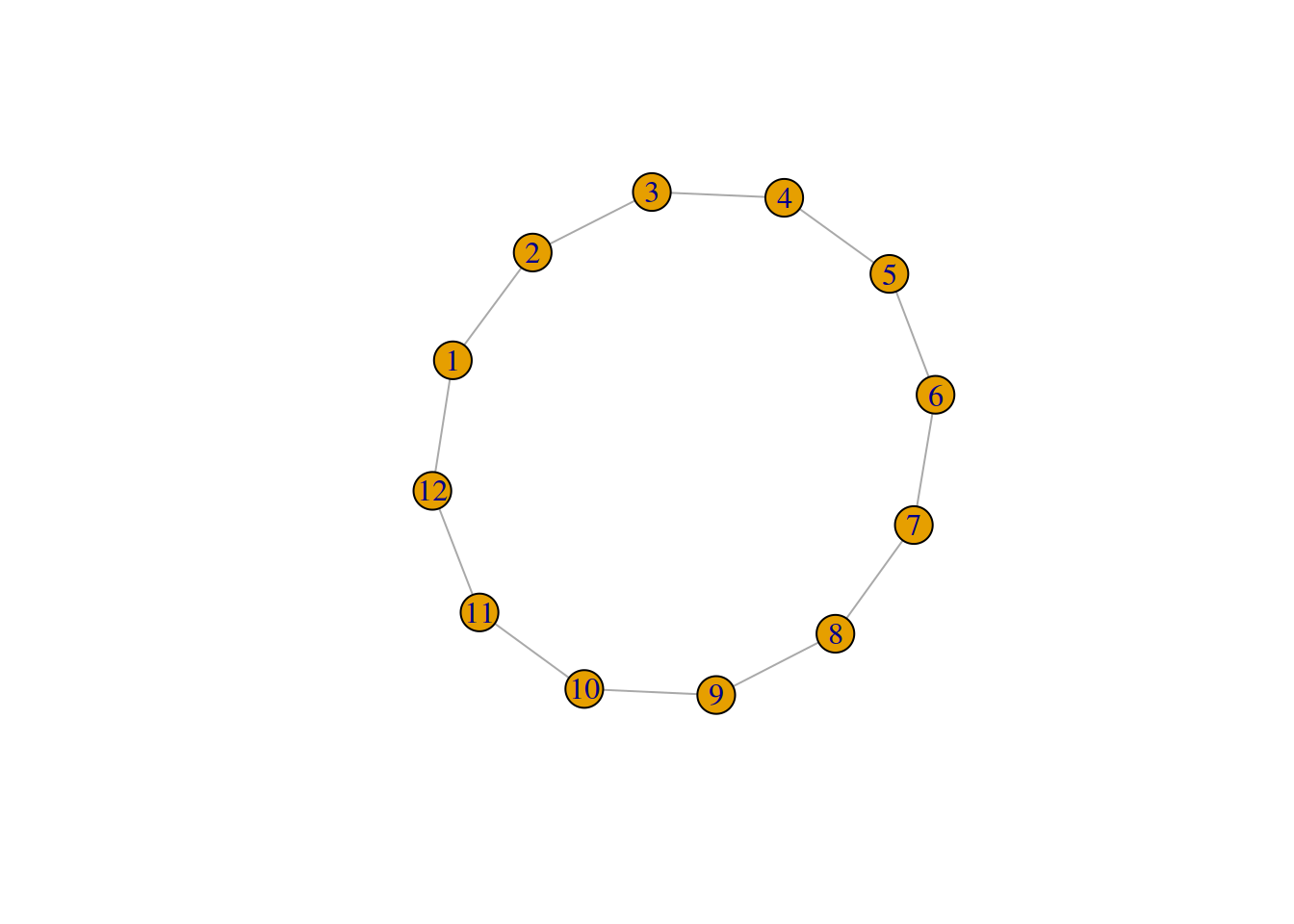As you see, the nodes of the graph above form a single cycle, a ring, and are numbered from 1 to 12.

You can generate other kinds of graphs, some of them with a random component. If you want to learn more, here is an additional tutorial on network models in R.

We are going to use as an example the social network of interactions between the characters of Star Wars Episode IV. You can find an example of the visualization of that network in my previous work about gender and networks in movie scripts and social media.

The dataset has two dataframes that we can use to get the information about nodes and the information about edges. We rename the columns of the interactions dataframe to fit the edges definition and we add an id to the characters dataframe to refer to characters by their node id:

``````charactersdf <- read.csv("Characters-IV.csv")
names(interactionsdf) <- c("to","from","weight") #for tbl_graph
charactersdf\$id <- seq(1:(nrow(charactersdf)))``````

The original dataset of movie characters has characters numbered from zero, but tidygraph (and most packages in R) have indices that start in 1. For that we add 1 to the entries of the edges table:

``````interactionsdf\$to <- interactionsdf\$to+1
interactionsdf\$from <- interactionsdf\$from+1``````

Now both dataframes are ready to build a graph. With the function tbl_graph, we can use both dataframes, telling it to build an undirected network with the parameter directed set to FALSE:

``````starwars<-tbl_graph(charactersdf,interactionsdf, directed=F)
starwars``````
``````## # A tbl_graph: 22 nodes and 60 edges
## #
## # An undirected simple graph with 2 components
## #
## # Node Data: 22 x 2 (active)
##   name           id
##   <fct>       <int>
## 1 R2-D2           1
## 2 CHEWBACCA       2
## 3 C-3PO           3
## 4 LUKE            4
## 6 CAMIE           6
## # … with 16 more rows
## #
## # Edge Data: 60 x 3
##    from    to weight
##   <int> <int>  <int>
## 1     1     2      3
## 2     1     3     17
## 3     1     9      1
## # … with 57 more rows``````

As you see, the result has a nodes part with the name of characters and their node id. The edges have endpoints in the columns “to” and “from” and a weight that counts the number of times those two characters interacted in the movie.

We can plot this network as we did with the ring graph:

``plot(starwars)``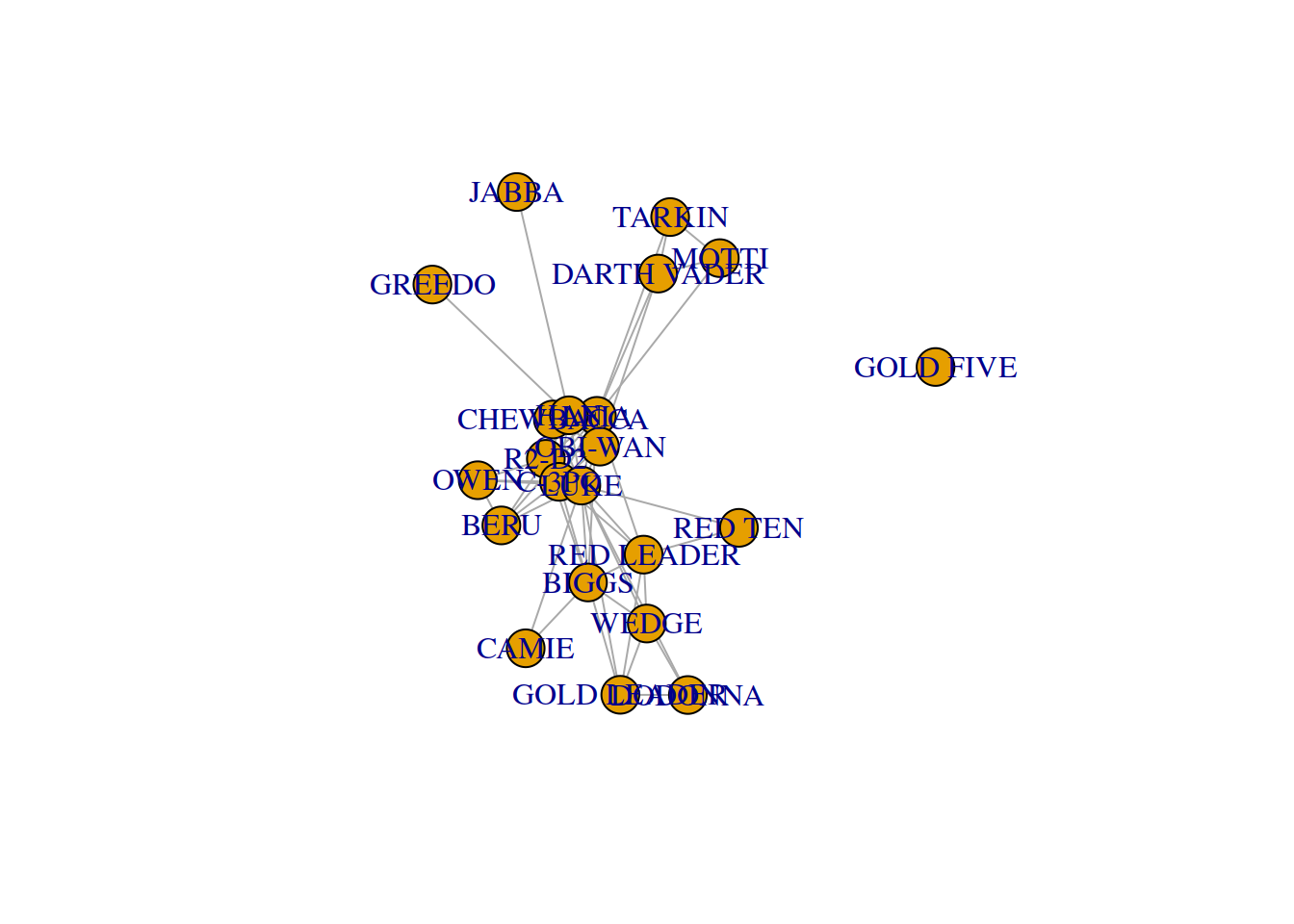Now nodes are labeled with the character name rather than a number as before. Depending on your resolution, you might be able to see some groups better than others, but ideally you should see the main characters making a dense community in the center. One character, “gold five”, has degree zero, as it talks in the movie but not clearly in a scene with another character.

### Handling network data

The data structure for networks in tidygraph contains various components that you might want to use in your analyses. Sometimes you want to do things with nodes, other times with edges. Tidygraph contains the function activate() for that, the result cab be converted to a tibble dataframe so you can analyze that data in particular:

``starwars %>% activate(nodes) %>% as_tibble()``
``````## # A tibble: 22 x 2
##    name           id
##    <fct>       <int>
##  1 R2-D2           1
##  2 CHEWBACCA       2
##  3 C-3PO           3
##  4 LUKE            4
##  6 CAMIE           6
##  7 BIGGS           7
##  8 LEIA            8
##  9 BERU            9
## 10 OWEN           10
## # … with 12 more rows``````
``starwars %>% activate(edges) %>% as_tibble()``
``````## # A tibble: 60 x 3
##     from    to weight
##    <int> <int>  <int>
##  1     1     2      3
##  2     1     3     17
##  3     1     9      1
##  4     1     4     14
##  5     1    10      1
##  6     1    11      4
##  7     1     8      5
##  8     1     7      1
##  9     1    14      6
## 10     2    11      4
## # … with 50 more rows``````

The result of activate() can be handled with the typical dplyr functions. For example here, we sort the edges by descending value of their weight and print the 6 with the highest weight:

``starwars %>% activate(edges) %>% arrange(desc(weight)) %>% as_tibble() %>% head()``
``````## # A tibble: 6 x 3
##    from    to weight
##   <int> <int>  <int>
## 1     4    14     26
## 2     2    14     19
## 3     4    11     19
## 4     3     4     18
## 5     1     3     17
## 6     4     8     17``````

Tidygraph allows you to get a particular column or variable from edges and nodes with the pull() function. For example here we take the weight out of the edges and plot their histogram:

``````starwars %>% activate(edges) %>% pull(weight) -> frequencies
hist(frequencies)``````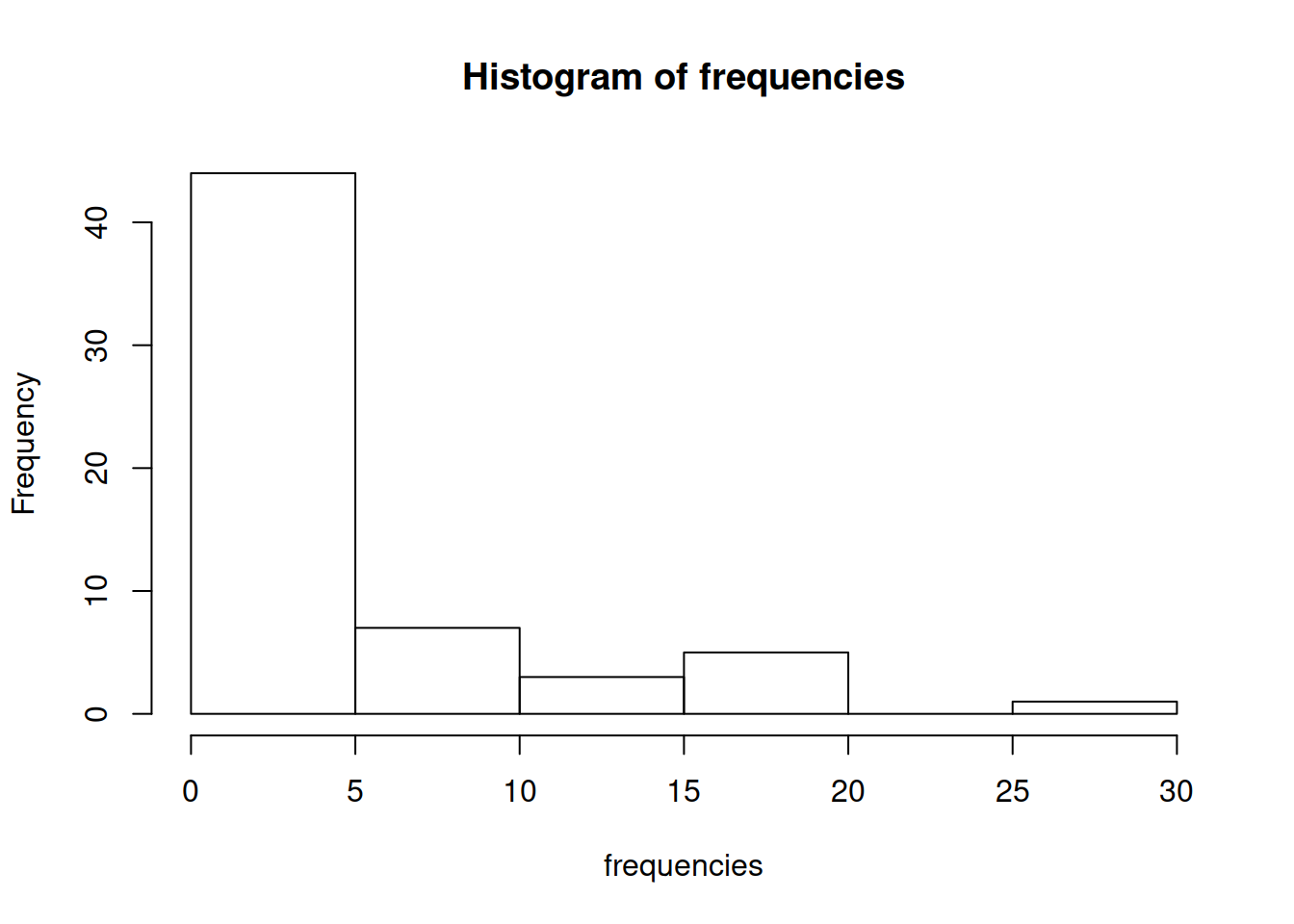You can do similar operations with other dplyr verbs. For example, here we first operate on the edges to remove the ones with weight lower than or equal to 10, then calculate node degree in the resulting network, and plot with the nodes that had degree larger than zero:

``starwars %>% activate(edges) %>% filter(weight>10) %>% activate(nodes) %>% mutate(degree=centrality_degree()) %>% filter(degree>0) %>% plot()``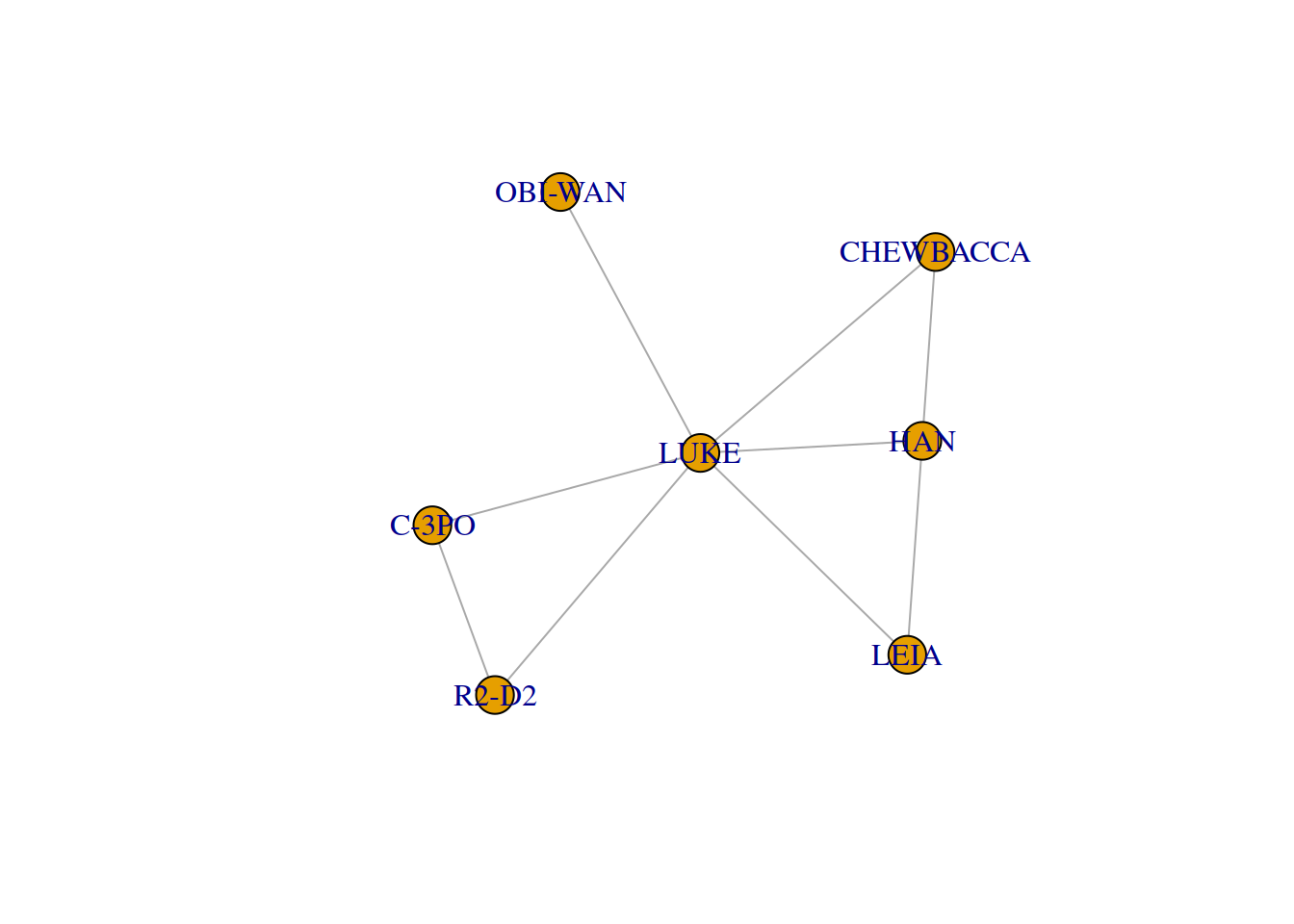Above we have visualized a kind of “network core” where only nodes connected to another node with sufficient weight are plotted.

Many calculations on a network depend on the neighbors of a node. The function local_members allows you to get neighborhoods at various distances, the first neighbors would be of order 1 but you can also get them for farther distances with higher orders. In the code below, we mutate each node to add its first-order neighbors and look at the neighborhood of the first:

``````starwars %>% activate(nodes) %>% mutate(neighborhood = local_members(mindist = 1, order=1))  %>% as_tibble() -> nodedata

nodedata\$neighborhood``````
``````## []
##   2  3  4  7  8  9 10 11 14``````

The result is a list of arrays with the ids of the nodes connected to the first. This structure is a bit complex because it was designed to have various arrays for different distances. If you want to get just the first array entry, you can use the index you want with double brackets “[[ ]]”:

``nodedata\$neighborhood[]``
``##    1  2  4  7  8  9 10 11 14 20``

Often, having node ids like above is not the most useful if we identify better nodes by their name. For example, we can get the name of the node with id 3:

``nodedata\$name``
``````##  R2-D2
## 22 Levels: BERU BIGGS C-3PO CAMIE CHEWBACCA DARTH VADER DODONNA ... WEDGE``````

And the naes of its neighbors by using the information que calculated above:

``nodedata\$name[nodedata\$neighborhood[]]``
``````##   R2-D2      CHEWBACCA  LUKE       BIGGS      LEIA       BERU
##   OWEN       OBI-WAN    HAN        RED LEADER
## 22 Levels: BERU BIGGS C-3PO CAMIE CHEWBACCA DARTH VADER DODONNA ... WEDGE``````

### Basic network visualization

It is good to make simple plots of the networks we handle as above, so we have an idea of how it looks like and that there are no obvious errors. The library ggraph is useful to plot graphs in a clearer way than just the plot() function that comes with tidygraph. It is based on the ggplot2 package, which is a useful package to make professional plots. We do not cover ggplot2 in this course, but you can learn more about it in this tutorial.

The basic call to ggraph() generates an object to plot. The geom_node_point() function tells that we want to plot nodes as solid points, and the geom_edge_link() function that we want to connect them with straight lines. Finally, the theme_graph() function tells that we are trying to plot a network with the above information, so it uses a layout algorithm to chose the position of the nodes:

``````library(ggraph)
starwars %>% ggraph() + geom_node_point() + geom_edge_link()  +  theme_graph()``````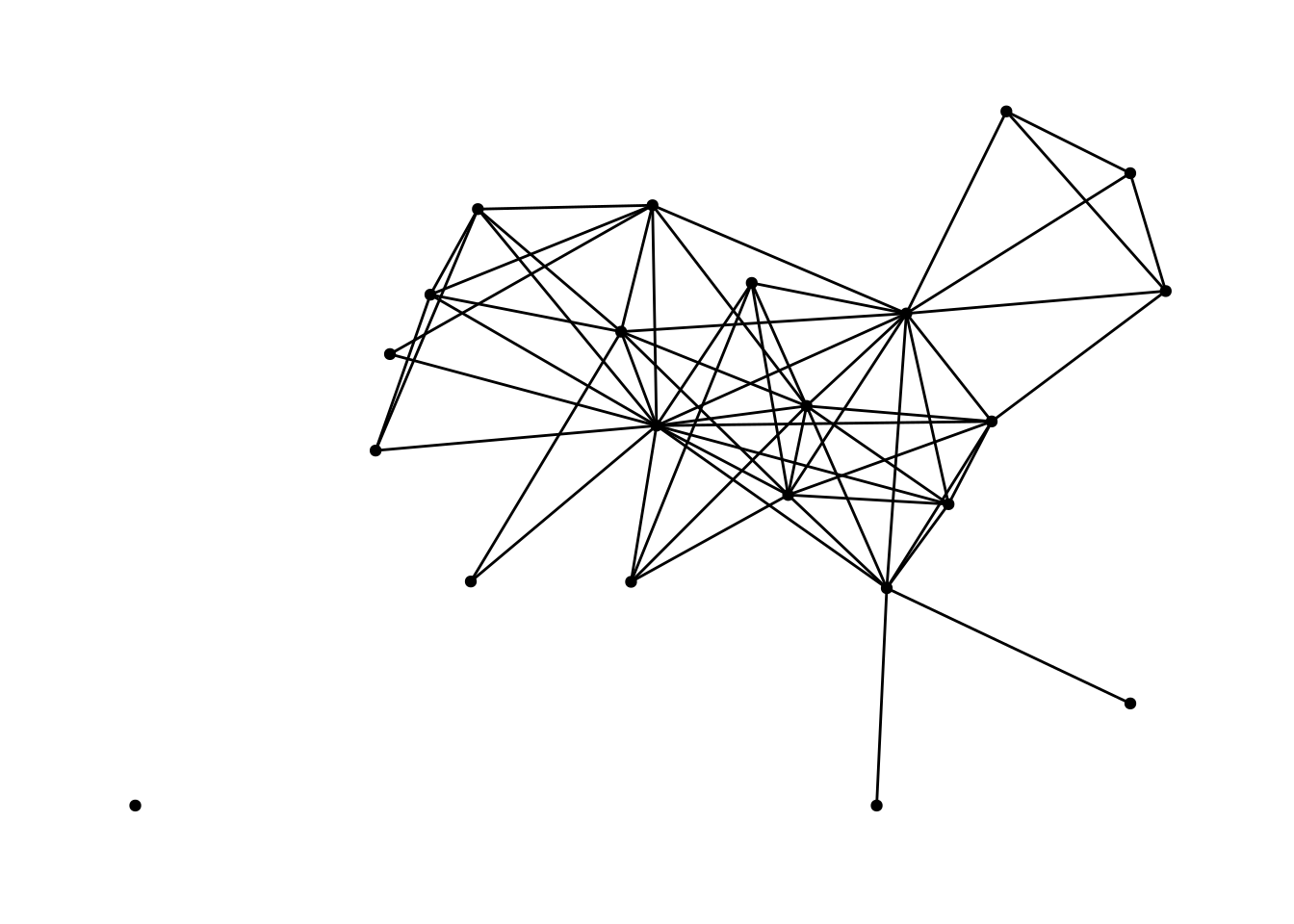Above you can get an idea of the network, but it is a very minimalistic plot without node names. You can add that by adding a “node text” with the geom_node_text() function. As a parameter it takes an aesthetics definition built with the function aes(), where we say that text labels should be the name variable of the node:

``starwars %>% ggraph() + geom_node_point() + geom_edge_link()  + geom_node_text(aes(label=name)) +  theme_graph()``
``## Using `stress` as default layout``